Applied and Computational Mathematics
Volume 5, Issue 3, June 2016, Pages: 103-106

On the Line Successive Overrelaxation Method

I. K. Youssef, Salwa M. Ali, M. Y. Hamada

Math. Dept., Faculty of Science, Ain Shams Uni., Cairo, Abbassia, Egypt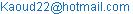(I. K. Youssef)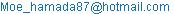(M. Y. Hamada)

I. K. Youssef, Salwa M. Ali, M. Y. Hamada. On the Line Successive Overrelaxation Method. Applied and Computational Mathematics. Vol. 5, No. 3, 2016, pp. 103-106. doi: 10.11648/j.acm.20160503.12

Received: May 17, 2016; Accepted: May 30, 2016; Published: June 13, 2016

Abstract: A line version of the KSOR method is introduced, LKSOR method. Comparison of the performance of some different iterative techniques with their line format (Jacobi – Gauss Seidel and SOR) are considered. Implementation of LKSOR method for several different formulas in different mesh geometries is discussed. The proposed method considers the advantages of the LSOR in addition to those of the KSOR. A graphical representation of the behavior of the spectral radius near the optimum value illustrates the smoothness in the selection of relaxation parameters.

Keywords: SOR, LSOR, KSOR Methods, Poisson Equation and Linear System

Contents

1. Introduction

The successive overrelaxation (SOR) method and its line variants [1,2,3] are among the most popular and efficient iterative methods used for solving large and sparse linear systems. Large linear systems appears in many areas of science and engineering, especially from the discretization of elliptic partial differential equations equation (3). The popularity of SOR algorithms is a great measure due to their simplicity from the programming and computational points of view, [4,5,6].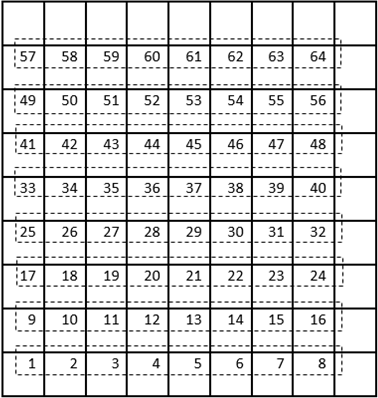Figure 1. Natural ordering of line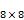mesh points.

The paper is devoted to describe a new version of efficient iterative algorithm for solving systems of linear equations, the line KSOR, LKSOR. The LKSOR method is adapted from the KSOR method by the same philosophy as done in the line successive overrelaxation method, LSOR, [1,3,4,5,6]. It is well- known that, the line Jacobi method performs like the point Gauss Seidel method for some classes of linear algebraic systems, [2,7,8,9].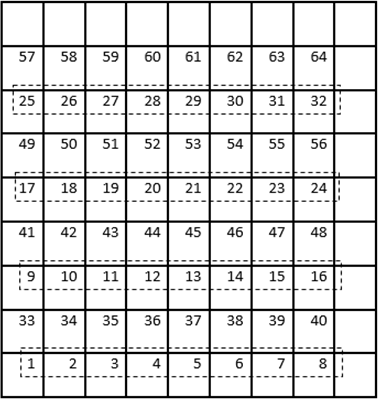Figure 2. Red black ordering of linemesh points.

In addition, the line Gauss Seidel performs faster than the point Gauss Seidel method. Moreover, the LSOR method can be adapted to give the same improvement done in the SOR, it is well known that the LSOR is asymptotically faster by a factor of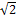over the point SOR, . Our main objective in this work is to introduce the line version of the KSOR method introduced in 2012,  as well as the comparison of its performance.

Implementation of LKSOR algorithms for several different grid labelling (natural and red black ordering) formulas in different mesh geometries are discussed. We also analyze the convergence behavior.

As usual, the discussion of the treatment is introduced through a well-known model problem, equation as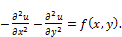(1)

Let, P denote a typical grid point of the grid defined in Figure (1) or Figure (2), R denote to the point to the right of the point P and L denote to the point to the left of the point P along the horizontal grid line similarly, T denote to the point above the point P and B denote to the point below the point P along the vertical grid line as shown in figure (3), [1,2].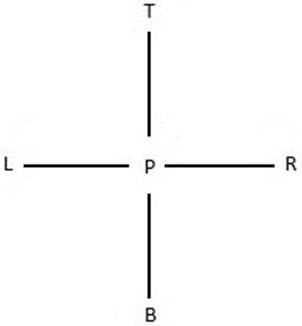Figure 3. Typical grid point.

Accordingly, equation (1) can be approximated at the point P by the equation,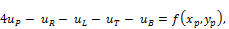(2)

this is known as the five point formula.

2. Line Iterative Methods

Partitioning large linear system into block forms is a problem in itself, in many cases the matrix of the smaller system has properties which make its solution particularly convenient, [2,10,11]. The treatment of partitioned systems is easier than the treatment of the overall system, since the subsystems are much smaller than the original system. Also, block iterative techniques is faster than the corresponding point wise form. In line iterative methods, one considers the linear systems obtained from the discretization of PDE over grids as described in Figure (1) or Figure (2). The unknowns are labeled as in the above figures, and the equations are collected along each grid line.

In addition, the partitioned system has properties, which the original system does not has. These properties, which the partitioned matrix has, very useful. For example, the 2-cyclic property of the coefficient matrix (14) which corresponding to Figure (1) enable one to obtain an eigenvalue functional relation (10) which can be used to calculate the ω optimum.

Consider a system of linear equations(3)

where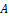is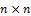matrix, is completely determined by a block partition of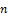-vectors into blocks. Specifically, suppose the-vector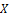is decomposed into sub-vectors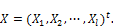(4)

In addition, each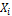is itself an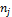-vectors associated with the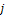points on the horizontal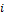line.

The line Jacobi iterative scheme is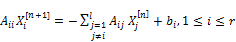(5)

moreover, the corresponding iteration matrix is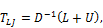(6)

where,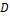is the block diagonal part,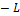is the block lower triangular part and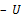is the block upper triangular part of the coefficient matrix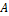.

Also, the line Gauss-Seidel scheme is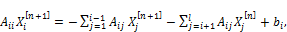(7)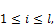the corresponding iteration matrix is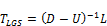(8)

While the line successive overrelaxation (LSOR) method with relaxation parameter ω is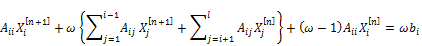and the corresponding iteration matrix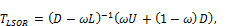(9)

where ω (0, 2) is a relaxation parameter. ω = 1, gives the line Gauss-Seidel method.

The rate of convergence of the SOR method depends on the choice of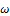. For certain classes of matrices (consistently ordered with property A), the optimum value can be obtained with the help of the eigenvalue functional relation Young: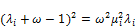(10)

where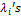are the eigenvalues of the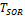the iteration matrix of the SOR method and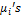are the eigenvalues of the Jacobi iteration matrix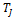.

Young  had illustrated that this functional relation still hold between eigenvalues of the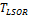iteration matrix and the eigenvalues of the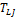iteration matrix.

Parter [7, 9, 10] shown that the line SOR method with optimum ω converges approximately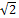as fast as the point SOR method.

3. Line KSOR Iterative Methods

Recently, Youssef , Youssef and Taha , Youssef and Al-Zaki , Youssef and Farid  and Youssef and Shukur , established a new forms of the SOR method, the KSOR. In the KSOR method, it is assumed that the current component can be used in the evaluation of the residue appears in the SOR method, in addition to the use the most recent calculated components used in the SOR. In the KSOR method the domain of the relaxation parameter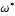is extended to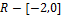instead of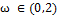in the SOR. The main objective of this work is to introduce the LKSOR and to compare its performance in comparison with the point as well as line iterative forms.

Using the same argument, the LKSOR method can be written in the form: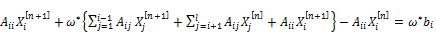(11)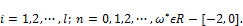or equivalently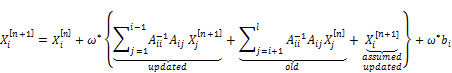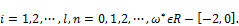The relaxation parameter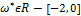plays the same role as ω in the SOR method.

The matrix formulation of the LKSOR method is: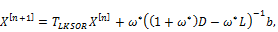(12)

where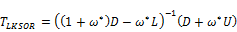(13)

Theorem (1):

The line KSOR iterative method is consistent.

Proof: Straightforward from formula (11).

Theorem (2):

The Line SOR and the Line KSOR satisfy the same eigenvalue functional relations as the corresponding point wise forms.

4. Numerical Calculation

We calculate the eigenvalues of the above mentioned iteration matrices, confirm the eigenvalue functional relations and represent graphically the behavior of the spectral radii. We consider a common linear system that arises from the discretization of Poisson’s equation: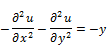with the initial condition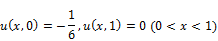and the boundary conditions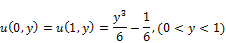If we use the five-point difference scheme as defined in (2) with grid spacing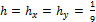and with a natural line ordering as shown in Figure (1), we obtain a linear system of equations with the structure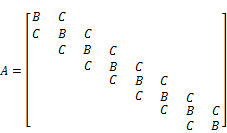(14)

where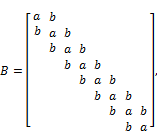and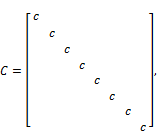where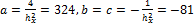.

The structure of the matrix A in the case of red-black as shown in Figure (2) ordering takes the form: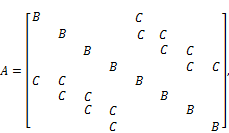with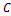and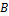still the same.

Then by applying variant line iterative methods (line Jacobi, line GS, LSOR, LKSOR) on the matrix (14), we get the results presented in Table (1).

Table 1. Comparison between variant line iterative methods.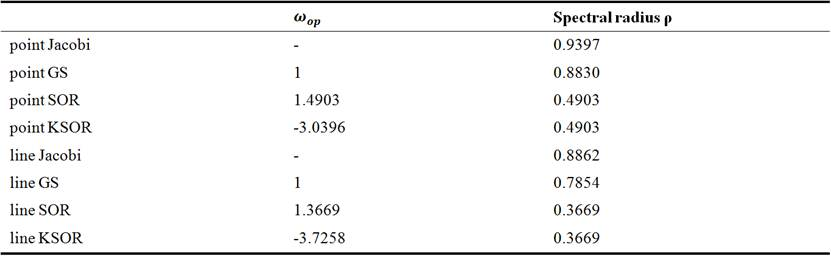Table (1) illustrates that, the convergence rate of the line J method is a relatively modest improvement as compared with the gain achieved in replacing the Jacobi method by the GS method; on the other hand, the line SOR method converges approximatelytimes as fast as the ordinary SOR method. Which satisfied also by line KSOR method. The behavior of the spectral radius of the line SOR and line KSOR are presented in Figure (4) and Figure (5) respectively.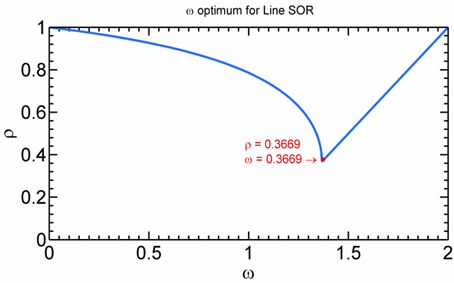Figure 4. The behavior of spectral radius of the line SOR.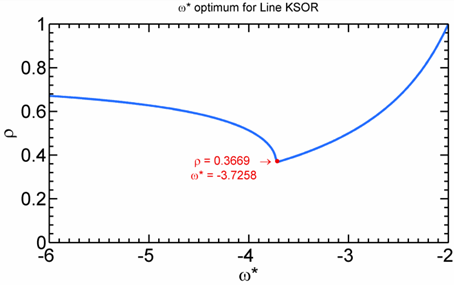Figure 5. The behavior of spectral radius of the line KSOR.

5. Conclusion

The LKSOR has the same simple structure as the KSOR. The behavior of the line methods preserves the same characteristics of block methods. It is faster than the point wise methods. The line red black ordering figure (2) give the same results as expected as the natural line ordering figure (1).

Table (1) confirms the results that the line versions of the iterative methods is faster than the corresponding point wise form. Moreover, it is clear that the performance of the LKSOR is the same as the LSOR and the LKSOR is faster than the KSOR by the same factor as LSOR method did with respect to SOR.

References

1. R. S. Varga, Matrix Iterative Analysis, Prentice-Hall, Englewood Cliffs, NJ, 1962.
2. D. M. Young, Iterative Solution of Large Linear Systems, Academic Press, New York, 1971.
3. Z. I. Woznicki, H. A. Jedrzejec, A new class of modified line-SOR algorithms, (2001), 89-142.
4. I. K. Youssef, "On the Successive Overrelaxation Method," Journal of Mathematics and Statistics 8 (2): 176-184, 2012.
5. R. J. Arms, L. D. Gates, and Zondek, B., A method of Block Iteration, J.Soc.Indust.App1.Math, (1956): Vol. 4, pp. 220-229.
6. I. K. Youssef, A. A. Taha, On the Modified Successive Overrelaxation method, Applied Mathematics and Computation, 219, 4601-4613, 2013.
7. S. V. Parter, Multi-line Iterative methods for Elliptic Difference Equations and Fundamental Frequencies, Numr. Math (1961):Vol. 3, pp.305-319.
8. I. K. Youssef, A. I. Alzaki, Minimization of l2-Norm of the KSOR Operator, Journal of Mathematics and Statistics, 2012.
9. S. V. Parter, Block Iterative methods, In Elliptic Problem Solvers, ed. Schultz, M. H., Academic Press, (1981): pp. 375-382.
10. S. V. Parter, Steuerwalt, M., Block Iterative method for Elliptic Finite Element Equation, Society for Industrial and Applied Mathematics, (1985):Vol. 22, No. 1.
11. D. J. Evans, M. J. Biggins, The Solution of Elliptic Partial Differential equation by a New Block Over-relaxation Technique, Intern. J. Computer. Math. (1982): 269–282.
12. I. K. Youssef, M. M. Farid, On the Accelerated Overrelaxation Method, Pure and Applied Mathematics Journal, 2015; 4(1): 26-31 .
13. I. K. Youssef, A. M. Shukur, Precondition for discretized fractional boundary value problem, Pure and Applied Mathematics Journal, 2014; 3(1): 1-6.

 Contents 1. 2. 3. 4. 5.
Article ToolsAbstractPDF(889K)